Given a binary search tree, print all possible arrays that produce the tree. An array produces a tree by inserting the array’s elements one by one into the tree. The elements are taken from the array from left to right.

Example. Consider the following binary search tree.

Its corresponding arrays are the following.

Input. The input file consists of positive integer N on the first line followed by N test cases. The first line of any given test case consists of a pair of positive integers M and R separated by a single space. Integer M indicates the number of edges in the binary search tree and R is the root of the tree. The M lines that remain in the test case consist of pairs of positive integers P and C corresponding to an edge that goes from parent node P to child node C. The following input file corresponds to the example.

Output. The output consists of all possible arrays for each test case. The arrays that correspond to a given test case must appear in lexicographical order. Each array must appear on a single line. To print an array, print the elements separated by space. For example, the corresponding is the output for the previous input.

Before you read our solution, try to solve the problem on your own. Remember that practice makes perfect.

2018-07-09 The Book of Problems currently supports Ruby, Python, Elixir, Java, C, OCaml, and now C++!

# Solution

We build each array from left to right by choosing for every position one node out of a set of possible choices for that position. The set of possible choices for a position is given by the corresponding array prefix. We illustrate the method with the following tree.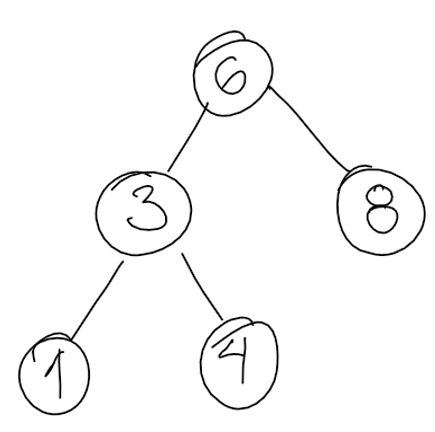We visit the tree starting from the root node. We create array $acc_0$. We collect the children of the root in set $cho_0$ as possible choices to visit next. The state is the following.We choose to visit node 3 next. We append 3 to the end of $acc_0$ and label the new array $acc_1$. We remove 3 from set of choices $cho_0$, add the children of 3, and label the new set as $cho_1$. The state is the following.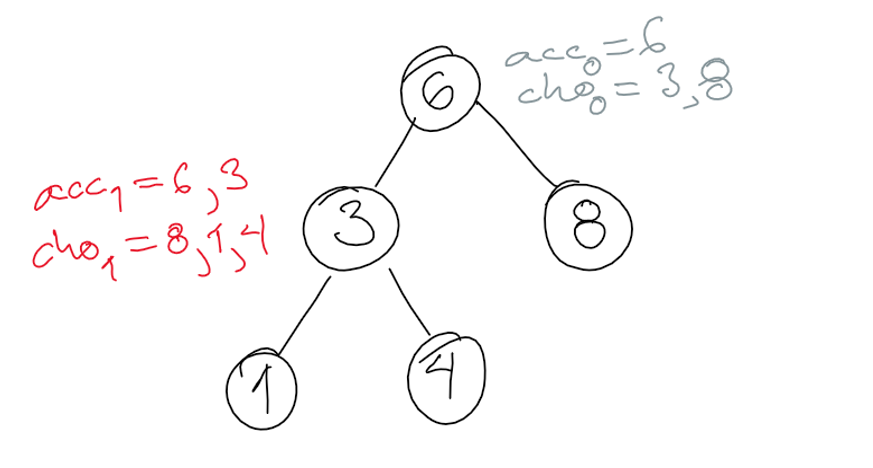We choose to visit node 8 next. We append 8 to the current array and label the result $acc_2$. We remove 8 from the set of choices and label the result $cho_2$. The state is the following.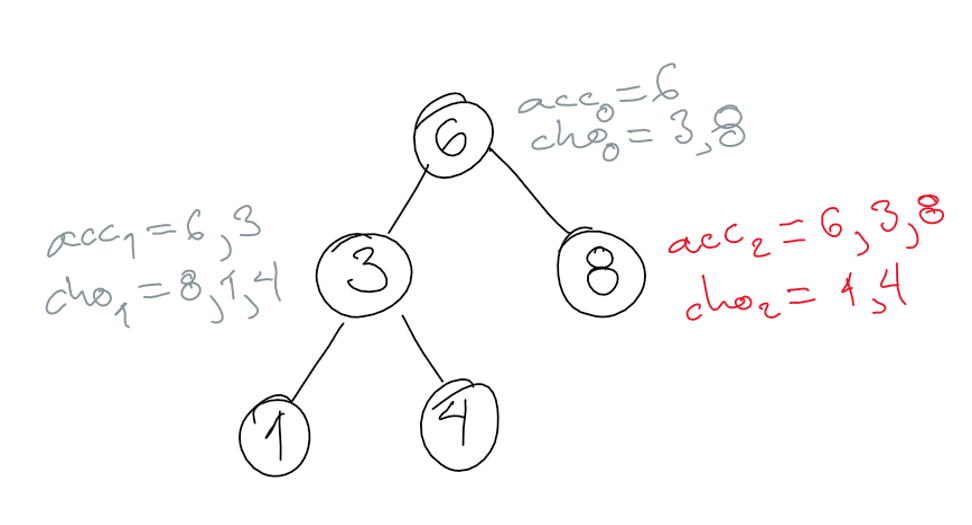We choose to visit node 1 next. The state is the following.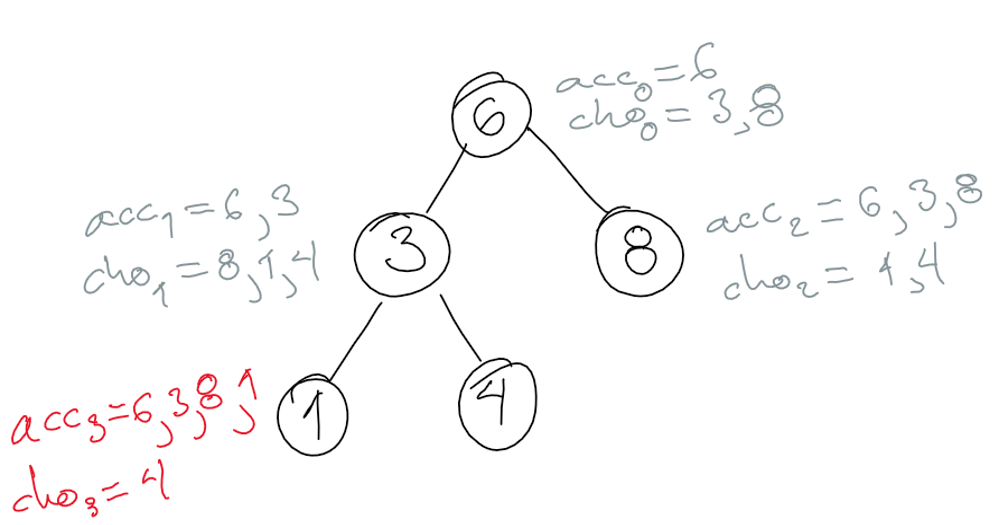We choose to visit node 4 next. There are no further choices. We have our first possible array. The state is the following.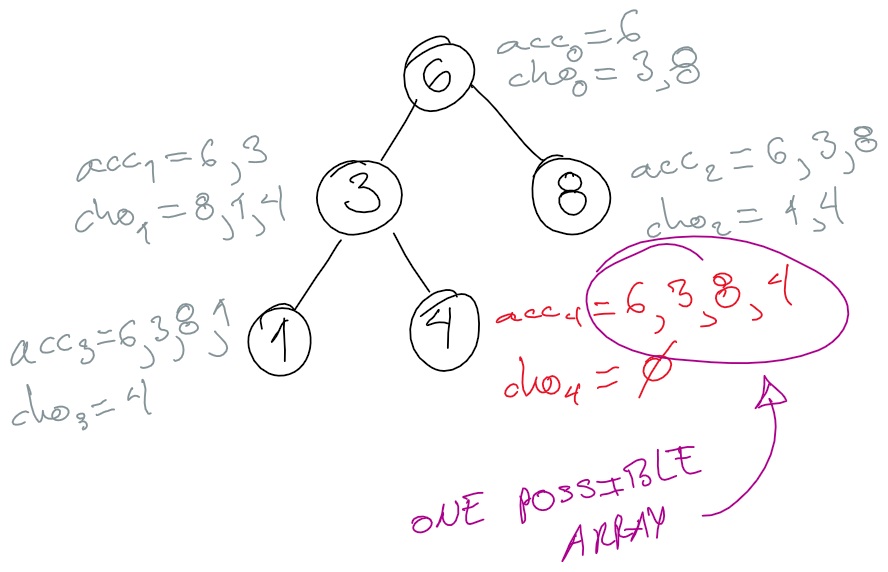To generate the rest of the possible arrays, we backtrack until we can make a different choice and apply the method again.

# Implementation

Here is a Ruby implementation.